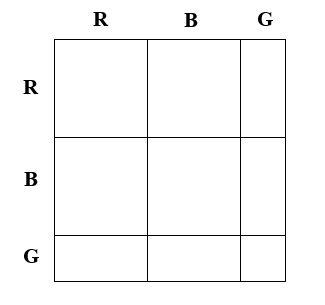Home > INT3 > Chapter 6 > Lesson 6.1.3 > Problem6-26

6-26.

In the casino game of roulette, some players think that if the ball lands on red several times in a row then it is more likely to land on black on the next spin. You can calculate probabilities to demonstrate that this is not the case. In other words, the outcome of one spin of a roulette wheel does not have any effect on the outcome of the next spin. Homework Help ✎

1. Make an area model for two spins of the wheel. There are $18$ red numbers, $18$ black numbers, and two green numbers.2. What is the probability that the ball will land on red twice in a row?

Use the diagram you made for part (a).

3. Based on your diagram, what is the probability that the ball will land on red on the second spin?

Use the diagram you made for part (a).

4. Calculate the conditional probability that the first spin was red given that the second spin is red.

Divide the probability you found in part (b) by the probability you found in part (c).

5. How do your answers to parts (c) and (d) compare to the probability of the ball landing on red for any single spin? If they are all the same, you have demonstrated that the probability of the ball landing on red is independent of where it landed before.

If they are not the same, check your work.